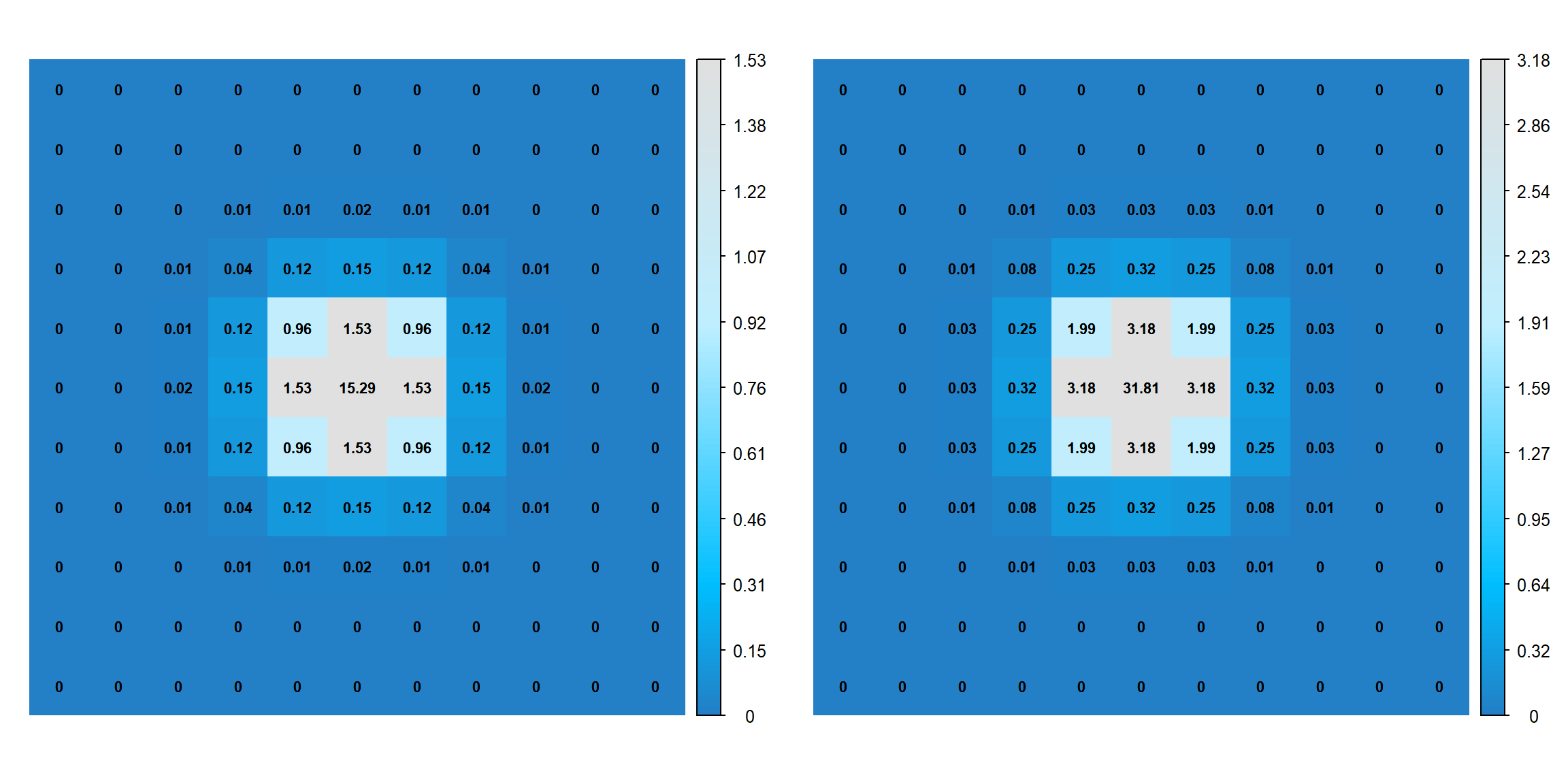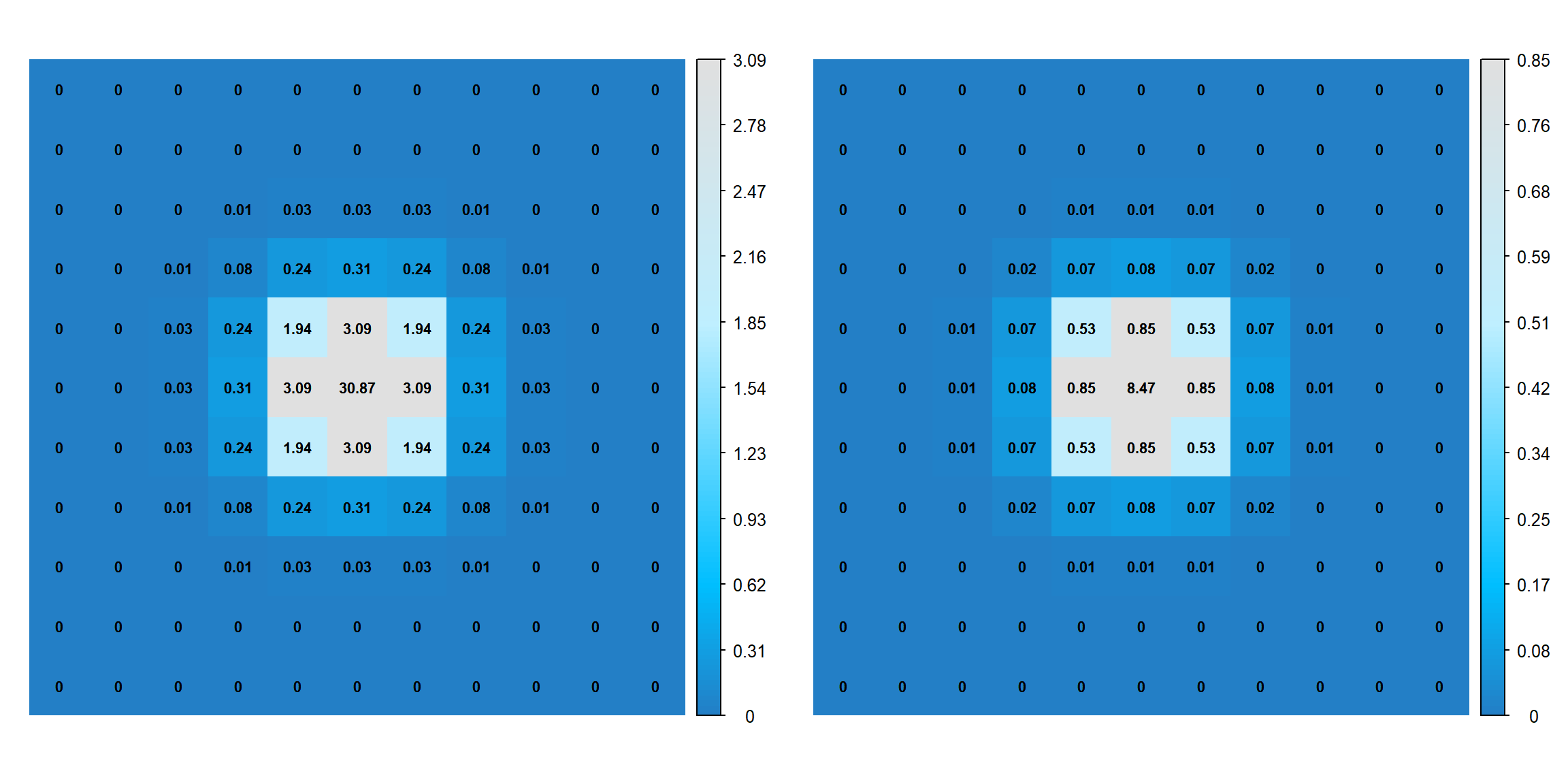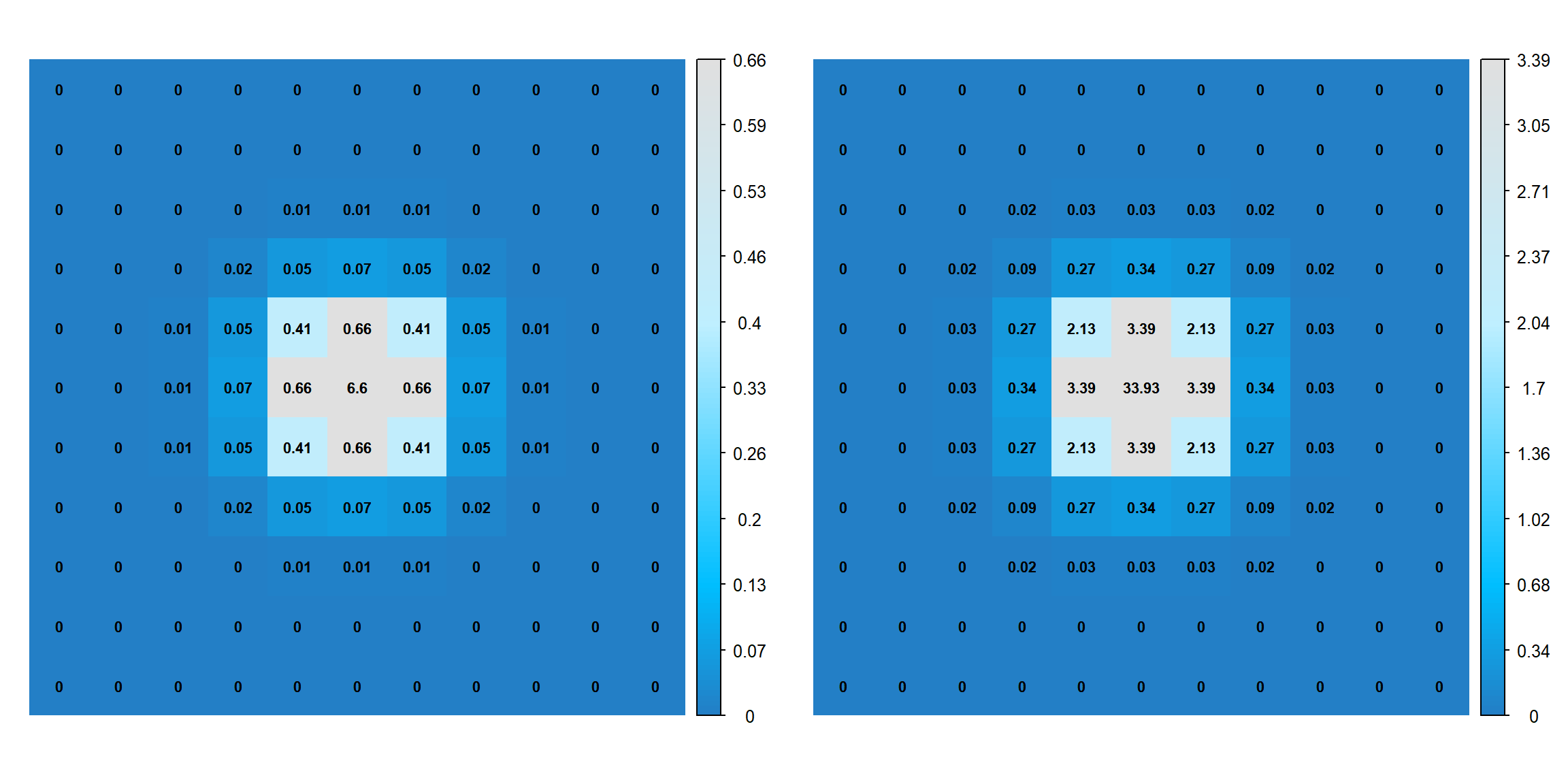Cellular Automata (CA) land-use models are widely used for understanding processes of land-use change. However, calibration of these models is a knowledge-intensive and time-consuming process. Although calibration of common driving factors such as accessibility (A), or suitability (S) is a relatively straightforward task, calibrating the neighbourhood dynamics (N), which controls the key model behaviour, is often very challenging. Here, building on the SIMLANDER modelling framework, we develop an automatic rule detection (ARD) procedure to automate the calibration of N. The process of ARD implementation includes:

## Step 1. Determination of the quantity and maximum size of moving windows

The initial requirement for automatic calibration of neighbourhood (N) is to define the “number of moving windows” (i.e. number of $$n$$ in $$N = [n_1, n_2, . n_n]$$) and their “maximum dimensions” that are required for the automatic calibration process.The former defined how many moving windows will be implemented and tested using ARD while the latter defined the initial dimensions of each constructed window.

##@@@@@@@@@@@@@@@@@@@@@@@@@@@@@@@@@
nw   <- 10 # How many initial matrix? 10, 50, 100?
size <- 11 # What should be the max dimension of each matrix? 11 stands for 11 by 11

## STEP 2. Setting up a matrix function

### STEP 2.1. Setting up a function to generate central value and decay rates

Then we need to set up a random Pythagorian Matrix function that is aimed at generating seed and drops that are central values of each matrix $$x_0,_0$$ and their corresponding decay rates $$\beta \$$, respectively. This function covers both random and grid options that can be further modified.

##@@@@@@@@@@@@@@@@@@@@@@@@@@@@@@@@@
randomPythagorianMatrix <- function(n, x, interpolation="smooth", search="random") {

choices <- c("random", "grid")
search  <- choices[pmatch(search, choices, duplicates.ok=FALSE)]
if(search=="random") {
seed   <- runif(n, 5, 50)
drops  <- mapply(runif, 1, 1, seed)
} else {
seed   <- rep(seq.int(5,n,5), each=5)
drops  <- rep(c(3,6,12,24,48), times=n/5)
}
Map(getPythagorianMatrix, x, seed, drops, interpolation)
}

### STEP 2.2. Setting up a function to generate random Pythagorian Matrix

Then we need to form a function that shapes an actual random Pythagorian Matrix using an smooth or linear interpolation as below:

##@@@@@@@@@@@@@@@@@@@@@@@@@@@@@@@@@
getPythagorianMatrix <- function(x, mid, drop, interpolation="smooth") {
if(x %% 2 == 0 | x < 0) stop("x must be an odd positive number")

choices <- c("smooth", "linear")
interpolation <- choices[pmatch(interpolation, choices, duplicates.ok=FALSE)]

dists <- outer(abs(1:x - ceiling(x/2)), abs(1:x - ceiling(x/2)), function(x,y) sqrt(x^2+y^2))
if(interpolation=="smooth") {
mat <- (1/drop) ^ dists * mid
} else {
mat <- matrix(approx(x=0:x, y=0.1^(0:x)*mid, xout=dists)$y, ncol=x, nrow=x) } return(mat) } ## STEP 3. Setting up plotPythagorianMatrix The plotPythagorianMatrix is used to plot all generated random Pythagorian matrices, where values of each cell is labled in the center. ##@@@@@@@@@@@@@@@@@@@@@@@@@@@@@@@@@ plotPythagorianMatrix <- function(mat) { colors <- colorRampPalette(c("deepskyblue4", "deepskyblue3", "darkslateblue", "deepskyblue1", "lightblue1", "gray88"))(256) corrplot::corrplot(mat, is.corr=FALSE, method="shade", col=colors, tl.pos="n", number.cex = .7, addCoef.col = "black" ) text(row(mat), col(mat), round(mat, 2), cex=0.7) } ## STEP 4. Setting up plotPythagorianMatrixDecay The decay rates are positive, pseudorandom number from the uniform distribution in the range $$0>\beta>1$$. For a randomised search, $$\beta \$$ may be a pseudorandom number from the uniform distribution, while for a grid search $$\beta \$$ may be any number from a user-defined set. Below function plots the resultant outcomes of applying each decay rate on every generated central cell. ##@@@@@@@@@@@@@@@@@@@@@@@@@@@@@@@@@ plotPythagorianMatrixDecay <- function(mat, plot=TRUE, ...) { mid <- ceiling(ncol(mat)/2) drop <- mat[mid, mid+1] / mat[mid, mid] xs <- seq(0, mid, 0.1) if(isTRUE(all.equal(mat[mid+1,mid+1], drop^sqrt(2) * mat[mid,mid]))) { ys <- drop^(xs)*mat[mid,mid] if(plot) { plot(xs, ys, type="l", las=1, lwd=2, xlab="distance", ylab="value", cex.axis=0.7, mgp=c(2,0.5,0), tck=-0.01, ... ) } } else { ys <- approx(0:mid, drop^(0:mid), xout=xs)$y * mat[mid,mid]
if(plot) {
plot(xs, ys, type="l", las=1, lwd=2, xlab="distance", ylab="value",
cex.axis=0.7, mgp=c(2,0.5,0), tck=-0.01, ...
)
}
}
invisible(data.frame(x=xs, y=ys))
}

## STEP 5. generating and plotting a matrix

### STEP 5.1. A linear interpolation

Below is an example Pythagorian matrix plot with the central value ($$x_0,_0$$) of 50 and decay rate ($$\beta \$$) of 5 using a linear interpolation:

#@@@@@@@@@@@@@@@@@@@@@@@@@@@@@@@@@
# SPECIFIED MATRICES
# Get a matrix with linear interpolation (excel)
mat1 <- getPythagorianMatrix(size, 50, 5, interpolation="linear")

par(mfrow=c(1,2))
plotPythagorianMatrix(mat1)
plotPythagorianMatrixDecay(mat1)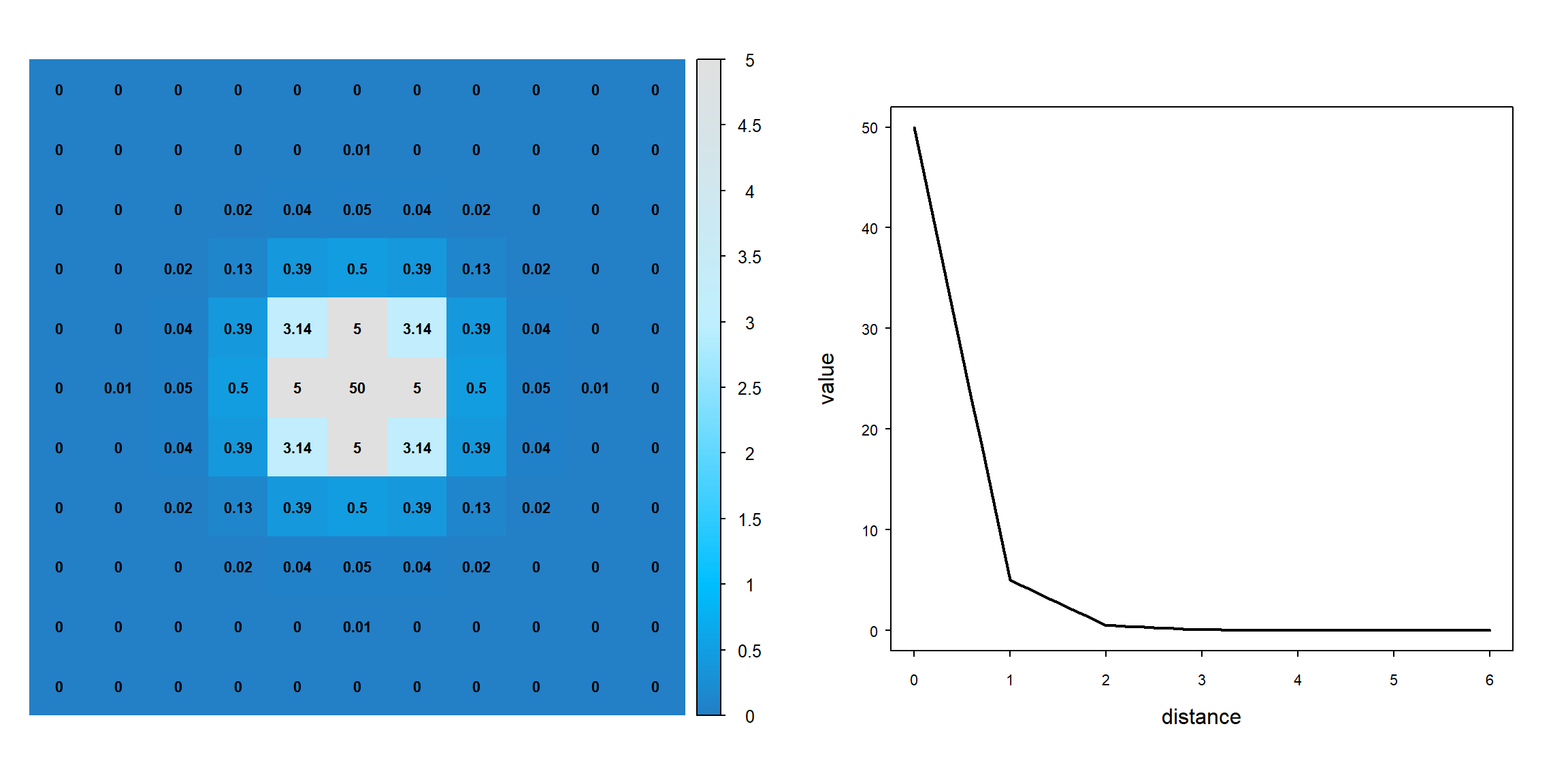### STEP 5.2. An smooth interpolation

Below is an example Pythagorian matrix plot with the central value ($$x_0,_0$$) of 50 and decay rate ($$\beta \$$) of 5 using an smooth interpolation:

#@@@@@@@@@@@@@@@@@@@@@@@@@@@@@@@@@
# SPECIFIED MATRICES
# Get a matrix with smooth interpolation (new proposal)
mat2 <- getPythagorianMatrix(size, 50, 5, interpolation="smooth")

par(mfrow=c(1,2))
plotPythagorianMatrix(mat2)
plotPythagorianMatrixDecay(mat2)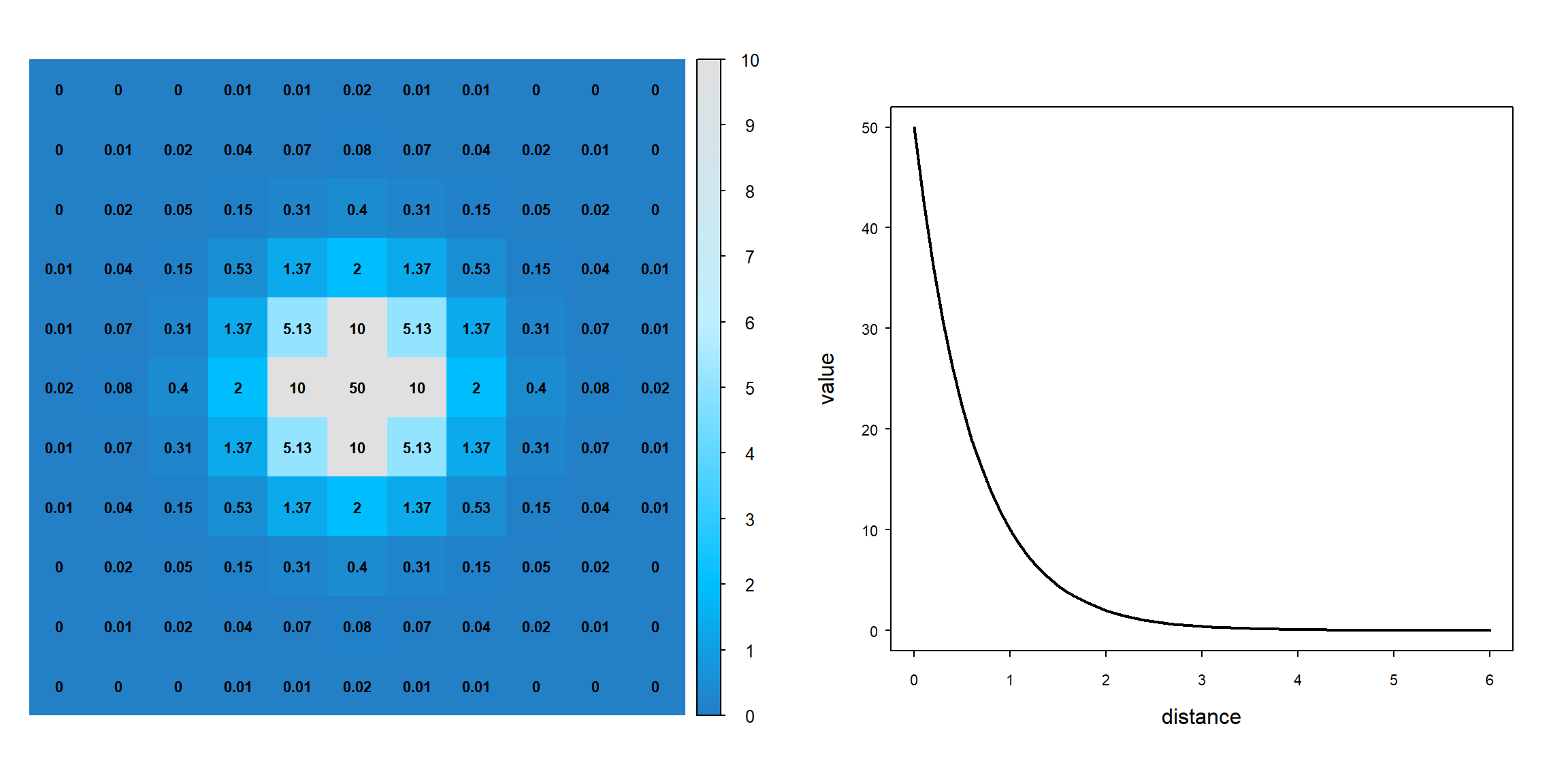### STEP 6. Generating desired number of random Pythagorian matrices

We then simply generate 10 matrix of $$11 * 11$$ dimension as below:

### STEP 6.1. Using linear function

#@@@@@@@@@@@@@@@@@@@@@@@@@@@@@@@@@
# RANDOM or GRID-BASED MATRICES
mats <- randomPythagorianMatrix(nw, size, interpolation="linear", search = "random")
#lapply(mats, plotPythagorianMatrix)
par(mfrow=c(1,2))
for (i in 1:10){
plotPythagorianMatrix(mats[[i]])
}